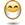# If certain number apply specific discount

## Excel Help for If Certain Number Apply Specific Discount in Analyzing Corporate Financial DataI have cell D4 were a user types in a number. If the number is equal to or less than cell J4 I want to apply the discount at J5, if the number is greater than J4 but less than cell K4 I want to apply the discount at K5, if the number is greater than K4 I want to apply the discount at L5. Anybody got a solutionThanks Posted by piper1509 on 22 Apr 2010Rate this: (3/5 from 1 vote) The formula for this is as follows:=IF(D4J4,D4